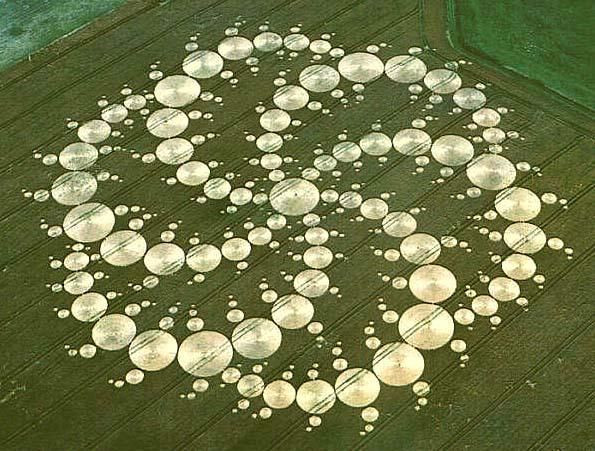Search

# Design a Crop Circle using Fibonacci Sequence

“Everything around you is mathematics!” Why confine our kids' minds to only a simple number series which is mathematically known as Natural Numbers. Let’s explore our view and try to recognize various kinds of numerical series whose illustrations we also see around us in nature. This activity will enable the child to learn a complex mathematical concept known as the Fibonacci Series in an easy to understand and tangible way.## Introduction:

Fibonacci numbers are an interesting mathematical idea. Fibonacci has a very evident prevalence of their appearance in nature and the ease of understanding them makes them an excellent principle to study.

A Fibonacci number sequence: 1, 1, 2, 3, 5, 8, 13 and so on. Every number in the sequence is the sum of the previous 2 numbers.

In this project, we will explore how can we design crop circles using the Fibonacci sequence.

### Mysterious Looking Crop Circles:Patterns generated by flattening the crop fields. These are more elaborate designs mainly using geometric forms. These have been appearing in grain fields all over the earth.

## Requirements:• Colored Paper

• Pencil and Ruler

• Compass

• Scissors

• Glue

## Steps:

1. Decide the size of the circle that you want to start with

2. Example: The first circle you decide to be ½ cm radius

Fibonacci Series of the same: ½, ½, 1, ½, 2 ½, 4, .....

2. Simply keep cutting the circles of the respective radii for the series

3. Once the circles are cut out, you can design your own corp circle

4. Arrange them in your way on a sheet and glue them accordingly

5. Share it with your friends

## Learning Opportunity:

• Mysteriously Appearing Corp Circles

• The Fibonacci numbers are a sequence of numbers in mathematics named after Leonardo of Pisa, known as Fibonacci

• Fibonacci Series: 0, 1, 1, 2, 3, 5, 8, 13, 21, 34, 55, 89, 144, …

• To get the next number in the sequence, we add the previous two numbers together

• For example 0+1=1 and 3+5=8. This sequence can go on forever

• Nature is full of Fibonacci numbers and the Fibonacci sequence

• Fibonacci Sequence well explains the spirals in pinecones, pineapples, daisies and lots of other things in nature

## Cost: NIL

#Middle #Maths #Fibonacci #CropCircle #STEAMProjects